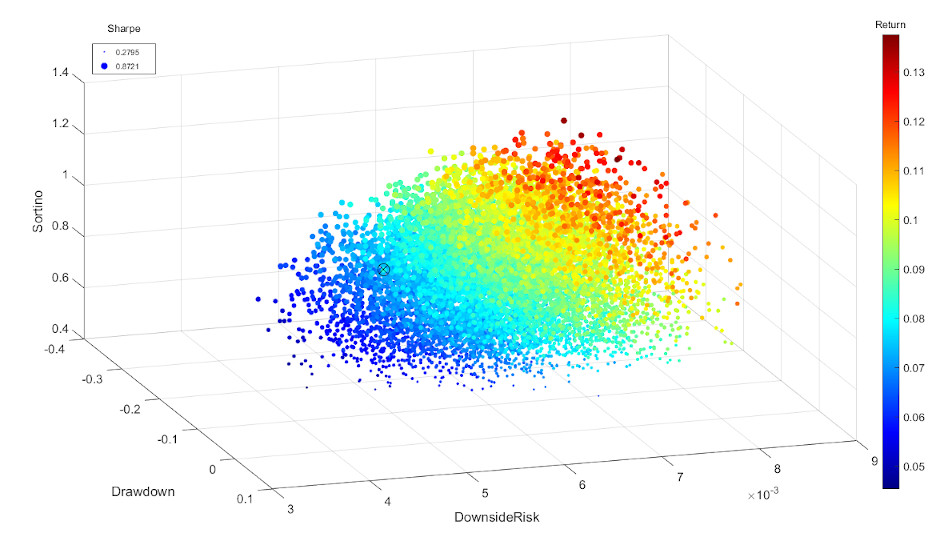Portfolio Sortino Ratio is an open-source Matlab toolbox for optimizing portfolio weights for a combination of metrics (Sortino Ratio, Total Return, Sharpe Ratio, Downside Risk, Max Drawdown)

### Investment portfolios with different mixes of stocks and bonds

Plot dimensions: Downside Risk, Max Drawdown, Sortino Ratio, Total Return, and Sharpe Ratio